## 5.44. balance_cycle

Origin
Constraint

$\mathrm{𝚋𝚊𝚕𝚊𝚗𝚌𝚎}_\mathrm{𝚌𝚢𝚌𝚕𝚎}\left(\mathrm{𝙱𝙰𝙻𝙰𝙽𝙲𝙴},\mathrm{𝙽𝙾𝙳𝙴𝚂}\right)$

Arguments
 $\mathrm{𝙱𝙰𝙻𝙰𝙽𝙲𝙴}$ $\mathrm{𝚍𝚟𝚊𝚛}$ $\mathrm{𝙽𝙾𝙳𝙴𝚂}$ $\mathrm{𝚌𝚘𝚕𝚕𝚎𝚌𝚝𝚒𝚘𝚗}\left(\mathrm{𝚒𝚗𝚍𝚎𝚡}-\mathrm{𝚒𝚗𝚝},\mathrm{𝚜𝚞𝚌𝚌}-\mathrm{𝚍𝚟𝚊𝚛}\right)$
Restrictions
 $\mathrm{𝙱𝙰𝙻𝙰𝙽𝙲𝙴}\ge 0$ $\mathrm{𝙱𝙰𝙻𝙰𝙽𝙲𝙴}\le \mathrm{𝚖𝚊𝚡}\left(0,|\mathrm{𝙽𝙾𝙳𝙴𝚂}|-2\right)$ $\mathrm{𝚛𝚎𝚚𝚞𝚒𝚛𝚎𝚍}$$\left(\mathrm{𝙽𝙾𝙳𝙴𝚂},\left[\mathrm{𝚒𝚗𝚍𝚎𝚡},\mathrm{𝚜𝚞𝚌𝚌}\right]\right)$ $\mathrm{𝙽𝙾𝙳𝙴𝚂}.\mathrm{𝚒𝚗𝚍𝚎𝚡}\ge 1$ $\mathrm{𝙽𝙾𝙳𝙴𝚂}.\mathrm{𝚒𝚗𝚍𝚎𝚡}\le |\mathrm{𝙽𝙾𝙳𝙴𝚂}|$ $\mathrm{𝚍𝚒𝚜𝚝𝚒𝚗𝚌𝚝}$$\left(\mathrm{𝙽𝙾𝙳𝙴𝚂},\mathrm{𝚒𝚗𝚍𝚎𝚡}\right)$ $\mathrm{𝙽𝙾𝙳𝙴𝚂}.\mathrm{𝚜𝚞𝚌𝚌}\ge 1$ $\mathrm{𝙽𝙾𝙳𝙴𝚂}.\mathrm{𝚜𝚞𝚌𝚌}\le |\mathrm{𝙽𝙾𝙳𝙴𝚂}|$
Purpose

Consider a digraph $G$ described by the $\mathrm{𝙽𝙾𝙳𝙴𝚂}$ collection. Partition $G$ into a set of vertex disjoint circuits in such a way that each vertex of $G$ belongs to a single circuit. $\mathrm{𝙱𝙰𝙻𝙰𝙽𝙲𝙴}$ is equal to the difference between the number of vertices of the largest circuit and the number of vertices of the smallest circuit.

Example
 $\left(\begin{array}{c}1,〈\begin{array}{cc}\mathrm{𝚒𝚗𝚍𝚎𝚡}-1\hfill & \mathrm{𝚜𝚞𝚌𝚌}-2,\hfill \\ \mathrm{𝚒𝚗𝚍𝚎𝚡}-2\hfill & \mathrm{𝚜𝚞𝚌𝚌}-1,\hfill \\ \mathrm{𝚒𝚗𝚍𝚎𝚡}-3\hfill & \mathrm{𝚜𝚞𝚌𝚌}-5,\hfill \\ \mathrm{𝚒𝚗𝚍𝚎𝚡}-4\hfill & \mathrm{𝚜𝚞𝚌𝚌}-3,\hfill \\ \mathrm{𝚒𝚗𝚍𝚎𝚡}-5\hfill & \mathrm{𝚜𝚞𝚌𝚌}-4\hfill \end{array}〉\hfill \end{array}\right)$ $\left(\begin{array}{c}0,〈\begin{array}{cc}\mathrm{𝚒𝚗𝚍𝚎𝚡}-1\hfill & \mathrm{𝚜𝚞𝚌𝚌}-2,\hfill \\ \mathrm{𝚒𝚗𝚍𝚎𝚡}-2\hfill & \mathrm{𝚜𝚞𝚌𝚌}-3,\hfill \\ \mathrm{𝚒𝚗𝚍𝚎𝚡}-3\hfill & \mathrm{𝚜𝚞𝚌𝚌}-1,\hfill \\ \mathrm{𝚒𝚗𝚍𝚎𝚡}-4\hfill & \mathrm{𝚜𝚞𝚌𝚌}-5,\hfill \\ \mathrm{𝚒𝚗𝚍𝚎𝚡}-5\hfill & \mathrm{𝚜𝚞𝚌𝚌}-6,\hfill \\ \mathrm{𝚒𝚗𝚍𝚎𝚡}-6\hfill & \mathrm{𝚜𝚞𝚌𝚌}-4\hfill \end{array}〉\hfill \end{array}\right)$ $\left(\begin{array}{c}4,〈\begin{array}{cc}\mathrm{𝚒𝚗𝚍𝚎𝚡}-1\hfill & \mathrm{𝚜𝚞𝚌𝚌}-2,\hfill \\ \mathrm{𝚒𝚗𝚍𝚎𝚡}-2\hfill & \mathrm{𝚜𝚞𝚌𝚌}-3,\hfill \\ \mathrm{𝚒𝚗𝚍𝚎𝚡}-3\hfill & \mathrm{𝚜𝚞𝚌𝚌}-4,\hfill \\ \mathrm{𝚒𝚗𝚍𝚎𝚡}-4\hfill & \mathrm{𝚜𝚞𝚌𝚌}-5,\hfill \\ \mathrm{𝚒𝚗𝚍𝚎𝚡}-5\hfill & \mathrm{𝚜𝚞𝚌𝚌}-1,\hfill \\ \mathrm{𝚒𝚗𝚍𝚎𝚡}-6\hfill & \mathrm{𝚜𝚞𝚌𝚌}-6\hfill \end{array}〉\hfill \end{array}\right)$

In the first example we have the following two circuits: $1\to 2\to 1$ and $3\to 5\to 4\to 3$. Since $\mathrm{𝙱𝙰𝙻𝙰𝙽𝙲𝙴}=1$ is the difference between the number of vertices of the largest circuit (i.e., 3) and the number of vertices of the smallest circuit (i.e., 2) the corresponding $\mathrm{𝚋𝚊𝚕𝚊𝚗𝚌𝚎}_\mathrm{𝚌𝚢𝚌𝚕𝚎}$ constraint holds.

All solutions

Figure 5.44.1 gives all solutions to the following non ground instance of the $\mathrm{𝚋𝚊𝚕𝚊𝚗𝚌𝚎}_\mathrm{𝚌𝚢𝚌𝚕𝚎}$ constraint: $\mathrm{𝙱𝙰𝙻𝙰𝙽𝙲𝙴}\in \left[0,1\right]$, ${S}_{1}\in \left[1,2\right]$, ${S}_{2}\in \left[1,3\right]$, ${S}_{3}\in \left[3,5\right]$, ${S}_{4}\in \left[3,4\right]$, ${S}_{5}\in \left[2,5\right]$, $\mathrm{𝚋𝚊𝚕𝚊𝚗𝚌𝚎}_\mathrm{𝚌𝚢𝚌𝚕𝚎}$$\left(\mathrm{𝙱𝙰𝙻𝙰𝙽𝙲𝙴},〈1{S}_{1},2{S}_{2},3{S}_{3},4{S}_{4},5{S}_{5}〉\right)$.

##### Figure 5.44.1. All solutions corresponding to the non ground example of the $\mathrm{𝚋𝚊𝚕𝚊𝚗𝚌𝚎}_\mathrm{𝚌𝚢𝚌𝚕𝚎}$ constraint of the All solutions slot; the $\mathrm{𝚒𝚗𝚍𝚎𝚡}$ attribute is displayed as indices of the $\mathrm{𝚜𝚞𝚌𝚌}$ attribute, and all vertices of a same cycle are coloured by the same colour.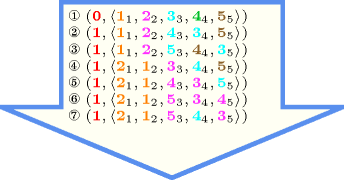Typical
$|\mathrm{𝙽𝙾𝙳𝙴𝚂}|>2$
Symmetry

Items of $\mathrm{𝙽𝙾𝙳𝙴𝚂}$ are permutable.

Arg. properties

Functional dependency: $\mathrm{𝙱𝙰𝙻𝙰𝙽𝙲𝙴}$ determined by $\mathrm{𝙽𝙾𝙳𝙴𝚂}$.

Counting
 Length ($n$) 2 3 4 5 6 7 8 9 10 Solutions 2 6 24 120 720 5040 40320 362880 3628800

Number of solutions for $\mathrm{𝚋𝚊𝚕𝚊𝚗𝚌𝚎}_\mathrm{𝚌𝚢𝚌𝚕𝚎}$: domains $0..n$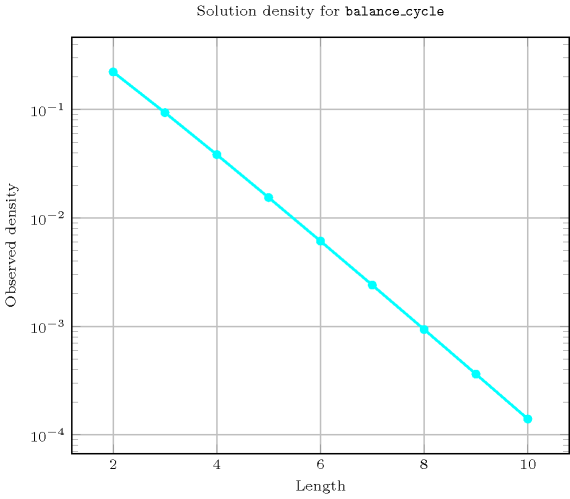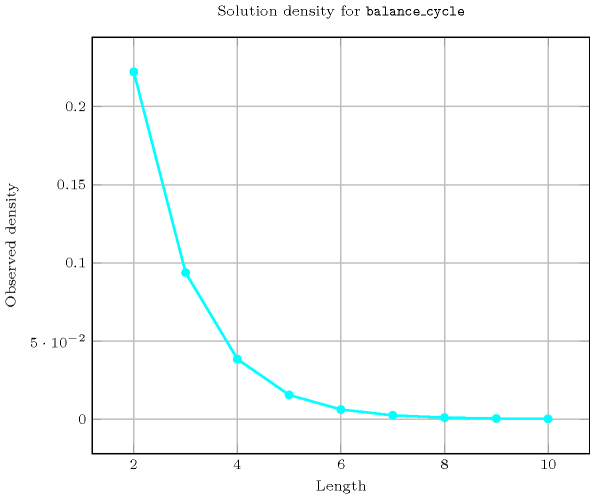Length ($n$)2345678910
Total26241207205040403203628803628800
 Parameter value

0231025176721640642561436402
1-36456086117782328384150
2--820250770798038808363680
3---30901344630075348456120
4----144504873645360708048
5-----840336066240378000
6------576025920572400
7-------45360226800
8--------403200

Solution count for $\mathrm{𝚋𝚊𝚕𝚊𝚗𝚌𝚎}_\mathrm{𝚌𝚢𝚌𝚕𝚎}$: domains $0..n$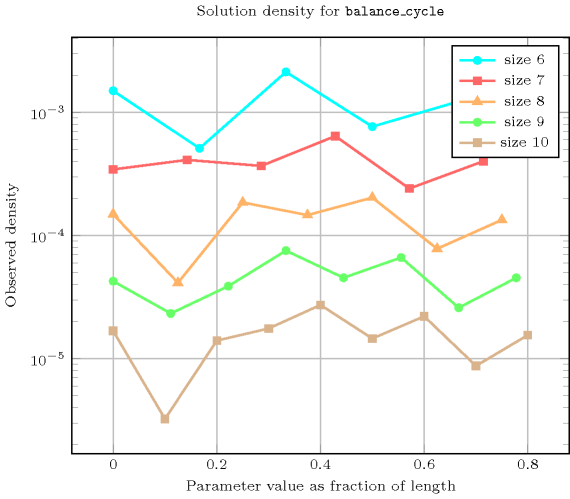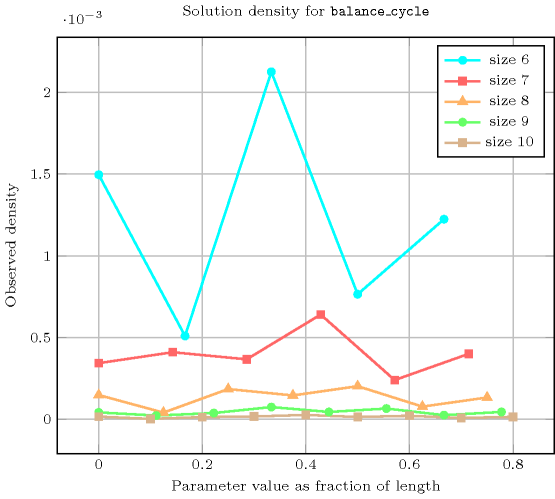related: $\mathrm{𝚋𝚊𝚕𝚊𝚗𝚌𝚎}$ (equivalence classes correspond to vertices in same cycle rather than variables assigned to the same value), $\mathrm{𝚌𝚢𝚌𝚕𝚎}$ (do not care how many cycles but how balanced the cycles are).

Keywords
Cond. implications

$•$ $\mathrm{𝚋𝚊𝚕𝚊𝚗𝚌𝚎}_\mathrm{𝚌𝚢𝚌𝚕𝚎}\left(\mathrm{𝙱𝙰𝙻𝙰𝙽𝙲𝙴},\mathrm{𝙽𝙾𝙳𝙴𝚂}\right)$

with  $\mathrm{𝙱𝙰𝙻𝙰𝙽𝙲𝙴}>0$

and   $\mathrm{𝙱𝙰𝙻𝙰𝙽𝙲𝙴}\le 2$

implies $\mathrm{𝚊𝚕𝚕}_\mathrm{𝚍𝚒𝚏𝚏𝚎𝚛}_\mathrm{𝚏𝚛𝚘𝚖}_\mathrm{𝚊𝚝}_\mathrm{𝚕𝚎𝚊𝚜𝚝}_𝚔_\mathrm{𝚙𝚘𝚜}$$\left(𝙺:\mathrm{𝙱𝙰𝙻𝙰𝙽𝙲𝙴},\mathrm{𝚅𝙴𝙲𝚃𝙾𝚁𝚂}:\mathrm{𝙽𝙾𝙳𝙴𝚂}\right)$.

$•$ $\mathrm{𝚋𝚊𝚕𝚊𝚗𝚌𝚎}_\mathrm{𝚌𝚢𝚌𝚕𝚎}\left(\mathrm{𝙱𝙰𝙻𝙰𝙽𝙲𝙴},\mathrm{𝙽𝙾𝙳𝙴𝚂}\right)$

implies $\mathrm{𝚙𝚎𝚛𝚖𝚞𝚝𝚊𝚝𝚒𝚘𝚗}$$\left(\mathrm{𝚅𝙰𝚁𝙸𝙰𝙱𝙻𝙴𝚂}:\mathrm{𝙽𝙾𝙳𝙴𝚂}\right)$.

Arc input(s)

$\mathrm{𝙽𝙾𝙳𝙴𝚂}$

Arc generator
$\mathrm{𝐶𝐿𝐼𝑄𝑈𝐸}$$↦\mathrm{𝚌𝚘𝚕𝚕𝚎𝚌𝚝𝚒𝚘𝚗}\left(\mathrm{𝚗𝚘𝚍𝚎𝚜}\mathtt{1},\mathrm{𝚗𝚘𝚍𝚎𝚜}\mathtt{2}\right)$

Arc arity
Arc constraint(s)
$\mathrm{𝚗𝚘𝚍𝚎𝚜}\mathtt{1}.\mathrm{𝚜𝚞𝚌𝚌}=\mathrm{𝚗𝚘𝚍𝚎𝚜}\mathtt{2}.\mathrm{𝚒𝚗𝚍𝚎𝚡}$
Graph property(ies)
 $•$$\mathrm{𝐍𝐓𝐑𝐄𝐄}$$=0$ $•$$\mathrm{𝐑𝐀𝐍𝐆𝐄}_\mathrm{𝐍𝐂𝐂}$$=\mathrm{𝙱𝙰𝙻𝙰𝙽𝙲𝙴}$

Graph class
$\mathrm{𝙾𝙽𝙴}_\mathrm{𝚂𝚄𝙲𝙲}$

Graph model

From the restrictions and from the arc constraint, we deduce that we have a bijection from the successor variables to the values of interval $\left[1,|\mathrm{𝙽𝙾𝙳𝙴𝚂}|\right]$. With no explicit restrictions it would have been impossible to derive this property.

In order to express the binary constraint that links two vertices one has to make explicit the identifier of the vertices. This is why the $\mathrm{𝚋𝚊𝚕𝚊𝚗𝚌𝚎}_\mathrm{𝚌𝚢𝚌𝚕𝚎}$ constraint considers objects that have two attributes:

• One fixed attribute $\mathrm{𝚒𝚗𝚍𝚎𝚡}$ that is the identifier of the vertex,

• One variable attribute $\mathrm{𝚜𝚞𝚌𝚌}$ that is the successor of the vertex.

The graph property $\mathrm{𝐍𝐓𝐑𝐄𝐄}$ $=$ 0 is used in order to avoid having vertices that both do not belong to a circuit and have at least one successor located on a circuit. This concretely means that all vertices of the final graph should belong to a circuit.

Parts (A) and (B) of Figure 5.44.2 respectively show the initial and final graph associated with the first example of the Example slot. Since we use the $\mathrm{𝐑𝐀𝐍𝐆𝐄}_\mathrm{𝐍𝐂𝐂}$ graph property, we show the connected components of the final graph. The constraint holds since all the vertices belong to a circuit (i.e., $\mathrm{𝐍𝐓𝐑𝐄𝐄}$ $=$ 0) and since $\mathrm{𝙱𝙰𝙻𝙰𝙽𝙲𝙴}$ $=$ $\mathrm{𝐑𝐀𝐍𝐆𝐄}_\mathrm{𝐍𝐂𝐂}$ $=$ 1.

##### Figure 5.44.2. Initial and final graph of the $\mathrm{𝚋𝚊𝚕𝚊𝚗𝚌𝚎}_\mathrm{𝚌𝚢𝚌𝚕𝚎}$ constraint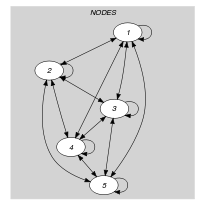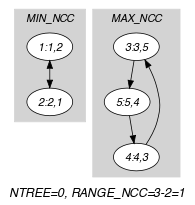(a) (b)